Elementary math Ratio and proportion

Converting F, D, and P

# Converting fractions, decimals and percentages

Here you will learn about comparing fractions, decimals, and percentages, including how to define a fraction, a decimal, and a percentage, convert between them, and compare and order them.

Students first learn about comparing fractions and decimals in the fifth grade and expand this knowledge to percents in the sixth and seventh grades when working with ratio and proportional relationships and the number system.

## What are fractions, decimals, and percentages?

Fractions, decimals, and percentages are different ways of expressing the same value.

• Fractions

Fractions equal parts of a whole.

The denominator of a fraction (number below the line) shows how many equal parts the whole has been divided into. The numerator of a fraction (number above the line) shows how many of the equal parts we have.

3 parts shaded out of 5 equal parts.

Step-by-step guide: Fractions

• Decimals

Decimals are numbers that have a whole part and a fractional part that are separated by a decimal point. Our decimal system splits whole numbers into tenths, hundredths, thousandths, and so on.

0.15 is represented on the place value chart.

Step-by-step guide: Decimals

• Percentages

Percentages are numbers which are expressed as a fraction of 100. Percent means “number of parts per hundred” and the symbol used for percent is, \% (percent sign).

42 shaded parts out of 100 equal parts is \, \cfrac{42}{100} \, which is 42\% .

Step-by-step guide: Percents

Let’s look at how you can express twenty-hundredths, \, \cfrac{20}{100} \, , as a fraction, decimal and percent.

### What is converting fractions, decimals, and percentages?

In order to compare fractions, decimals and percentages, you will need to know how to convert them to the same form.

Step-by-step guide: Decimal to a percent

Step-by-step guide: Fraction to a percent

Step-by-step guide: Powers of 10

Step-by-step guide: Fraction to a decimal

Step-by-step guide: Percent to a decimal

### Comparing fractions, decimals, and percentages

Now that you know how to convert fractions, decimals, and percentages to the same form, let’s compare them.

For example, list the following numbers in order from least to greatest.

\cfrac{1}{4} \hspace{0.5cm} 0.19 \hspace{0.5cm} 0.3 \hspace{0.5cm} 26 \% \hspace{0.5cm} \cfrac{1}{5}

Before comparing the numbers, convert them all to fractions with a denominator of 100.

\cfrac{1}{4}=\cfrac{1 \, \times \, 25}{4 \, \times \, 25}=\cfrac{25}{100}

0.19=\cfrac{19}{100}

0.3=\cfrac{3}{10}=\cfrac{3 \, \times \, 10}{10 \, \times \, 10}=\cfrac{30}{100}

26 \%=\cfrac{26}{100}

\cfrac{1}{5}=\cfrac{1 \, \times \, 20}{5 \, \times \, 20}=\cfrac{20}{100}

The numbers in order from least to greatest:

0.19=\cfrac{19}{100} \, , \; \cfrac{1}{5}=\cfrac{20}{100} \, , \; \cfrac{1}{4}=\cfrac{25}{100} \, , \; 26 \%=\cfrac{26}{100} \, , \; 0.3=\cfrac{30}{100}

0.19, \; \cfrac{1}{5} \, , \; \cfrac{1}{4} \, , \; 26 \%, \; 0.3

Another way you could have done the comparison is to convert all the numbers to percents.

\cfrac{1}{4}=\cfrac{25}{100}=25 \%

0.19 \, → multiply by 100 to change a decimal to a percent.

0.19 \times 100=19. This is the same as moving the decimal point 2 decimal places to the right.

0.19=19 \%

0.3 \, → multiplied by 100 changes a decimal to a percent.

0.3 \times 100=30. This is the same as moving the decimal point 2 decimal places to the right.

0.3=30 \%

\cfrac{1}{5}=\cfrac{20}{100}=20 \%

The numbers from least to greatest is:

0.19=19 \%, \; \cfrac{1}{5}=20 \% \, , \; \cfrac{1}{4}=25 \%, \; 26 \%, \; 0.3=30 \%

0.19, \; \cfrac{1}{5} \, , \; \cfrac{1}{4} \, , \; 26 \%, \; 0.3

### What are fractions, decimals and percents?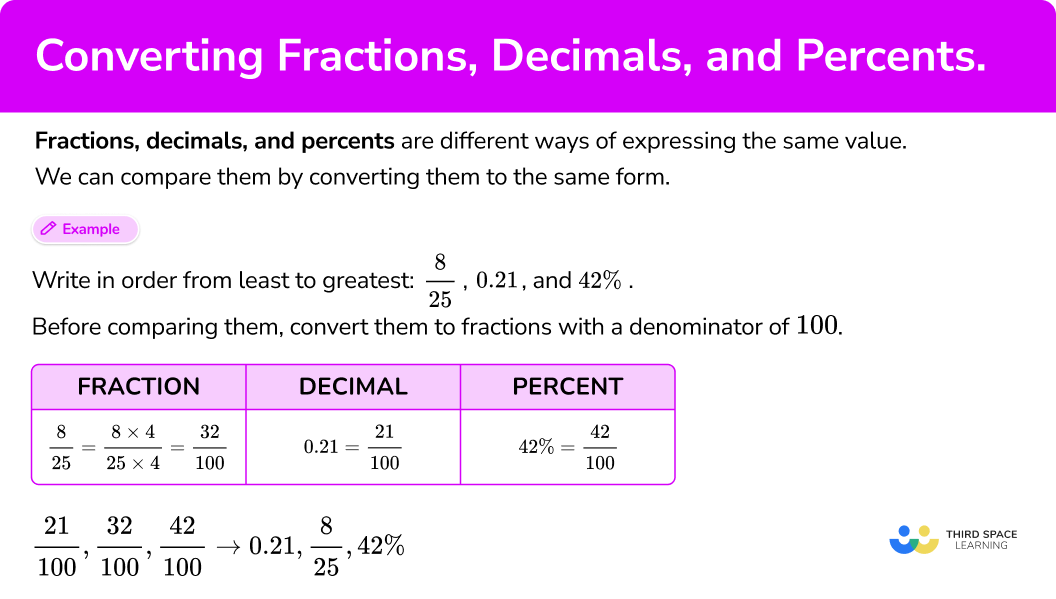## Common Core State Standards

How does this relate to 5th grade math and 6th grade math?

• Grade 5 – Number and Operations – Base Ten (5.NBT.A.3.a)
Read and write decimals to thousandths using base-ten numerals, number names, and expanded form, for example,
347.392 = 3 \times 100 + 4 \times 10 + 7 \times 1 + 3 \times \bigl(\cfrac{1}{10}\bigr) + 9 \times \bigl(\cfrac{1}{100}\bigr) + 2 \times \bigl(\cfrac{1}{1000}\bigr).

• Grade 6 – Ratios and Proportional Relationships (6.RP.A.3.c)
Find a percent of a quantity as a rate per 100 (for example, 30\% of a quantity means \; \cfrac{30}{100} \; times the quantity); solve problems involving finding the whole, given a part and the percent.

• Grade 7 – The Number System (7.NS.2d)
Convert a rational number to a decimal using long division; know that the decimal form of a rational number terminates in 0s or eventually repeats.

## How do you convert fractions, decimals, and percentages?

In order to compare fractions, decimals, and percentages, convert them to the same form.

1. Choose a form (fraction, decimal, percentage).
2. Convert the numbers to the form you chose.
3. Write the numbers in the new form. If you’re comparing the numbers, go to Step 4.
4. Write the numbers in the correct order.

## Converting fractions, decimals, and percentages examples

### Example 1: convert fraction, decimal, and percentage to the same form

Convert the numbers below to fractions with a denominator of 100.

\cfrac{3}{4} \, , \; 75 \%, \; 0.75

1. Choose a form (fraction, decimal, percentage).

Convert the numbers to fractions.

2Convert the numbers to the form you chose.

Since you are converting the numbers to fractions, use equivalent fractions to convert the denominator of the fraction to 100.

\cfrac{3}{4}= \cfrac{3 \, \times \, 25}{4 \, \times \, 25}= \cfrac{75}{100}

\cfrac{3}{4}= \cfrac{75}{100}

Write the decimal as a fraction.

0.75 is seventy-five hundredths, which is \, \cfrac{75}{100} .

Write the percent as a fraction.

75 \% is \, \cfrac{75}{100} \, .

3Write the numbers in the new form. If you’re comparing the numbers, go to Step 4.

\cfrac{3}{4}= \cfrac{75}{100}

0.75= \cfrac{75}{100}

75 \% =\cfrac{75}{100}

You are not comparing the numbers, so you do not have to go to Step 4.

### Example 2: convert fraction, decimal and percentage to a decimal

Convert the numbers below to decimals.

\cfrac{2}{5} \, , \; 40 \%

Convert the numbers to decimal numbers.

Rewrite the fraction as a division problem, then use long division to divide the numerator by the denominator.

2 \div 5

2 \div 5=0.4

Divide the percent number by 100.

40 \% =\cfrac{40}{100} = {40}\div 100

Recall from dividing by powers of 10, you can simply move the decimal point two places to the left when dividing by 100.

\cfrac{2}{5}=0.40=0.4

40 \%=\cfrac{40}{100} = 0.40 = 0.4

You are not comparing the numbers, so do not need to go to Step 4.

### Example 3: convert fraction, decimal, and percentage to decimals

Convert the numbers below to decimals.

\cfrac{2}{9} \, , \; 67 \%

Convert the numbers to decimal numbers.

Divide the numerator by the denominator using long division \, \cfrac{2}{9} = 2\div 9

2 \div 9=0 . \overline{2}

Make the percent a decimal by dividing the percent by 100 which is the same as moving the decimal point two places to the left.

67\%=\cfrac{67}{100} = 67\div 100

Using the rules of dividing by powers of ten 67 \div 100=0.67

\cfrac{2}{9} = 0.\overline{2}

0.\overline{2} rounded to the nearest hundredth is 0.22

67\% = 0.67

You are not comparing the numbers, so you do not have to go to Step 4.

### Example 4: compare fractions, decimals, and percentage by converting them to fractions

Write the numbers in order from least to greatest.

\cfrac{1}{2} \, , \; 0.24,33 \%, \; \cfrac{7}{20}

Change all the numbers to fractions with a denominator of 100.

Change \, \cfrac{1}{2} \, and \, \cfrac{7}{20} \, so they have a denominator of 100.

\cfrac{1}{2}=\cfrac{1 \, \times \, 50}{2 \, \times \, 50}=\cfrac{50}{100}

\cfrac{7}{20}=\cfrac{7 \, \times \, 5}{20 \, \times \, 5}=\cfrac{35}{100}

0.24 is twenty-four hundredths so can be written as \, \cfrac{24}{100} .

33\% can be written as the fraction \, \cfrac{33}{100} .

The numbers as fractions are:

\cfrac{1}{2}=\cfrac{50}{100}

\cfrac{7}{20}=\cfrac{35}{100}

0.24=\cfrac{24}{100}

33\%=\cfrac{33}{100}

The numbers in order from least to greatest are:

\cfrac{24}{100} \, , \; \cfrac{33}{100} \, , \; \cfrac{35}{100} \, , \; \cfrac{50}{100} \, which is 0.24, \; 33\%, \; \cfrac{7}{20} \, , \; \cfrac{1}{2}

### Example 5: compare fractions, decimals, and percentage by changing them all to percentages

Write the numbers in order from greatest to least.

45\% , \; 0.32, \; \cfrac{1}{4} \, , \; \cfrac{2}{5} \, , \; 0.18

Change all the numbers to percents.

Rewrite the fractions with a denominator of 100.

\cfrac{1}{4} \; \rightarrow \; \cfrac{1}{4}=\cfrac{1 \, \times \, 25}{4 \, \times \, 25}=\cfrac{25}{100}

\cfrac{2}{5} \; \rightarrow \; \cfrac{2}{5}=\cfrac{2 \, \times \, 20}{5 \, \times \, 20}=\cfrac{40}{100}

\cfrac{25}{100}=25\%

\cfrac{40}{100}= 40\%

Multiply the decimals by 100 or move the decimal point two places to the right.

0.32 \; \rightarrow \; 0.32 \times 100=32 \; \rightarrow \; 32 \%

0.18 \; \rightarrow \; 0.18 \times 100=18 \; \rightarrow \; 18 \%

45\%

\cfrac{40}{100}= 40\%

0.32= 32\%

\cfrac{25}{100}=25\%

0.18 = 18\%

The numbers in order from greatest to least are:

45 \%, \, 40 \%, \, 32 \%, \, 25 \%, \, 18 \% \; \rightarrow \; 45 \%, \, \cfrac{2}{5} \, , \, 0.32, \, \cfrac{1}{4} \, , \, 0.18

### Example 6: compare fractions, decimals, and percentage by changing them all to decimals

Write the numbers in order from least to greatest.

78 \%, \; 0.9, \; \cfrac{2}{3} \, , \; 61 \%

Change all the numbers to decimals.

Divide the numerator by the denominator using long division, \, \cfrac{2}{3} \, is 2 \div 3

2 \div 3=0 . \overline{6} which is a repeating decimal.

0 . \overline{6}=0.66666\ldots

0.66666\ldots Rounded to the nearest hundredth is 0.67

To change the percents to decimals, divide the percents by 100 or move the decimal point two places to the left.

78 \%=\cfrac{78}{100}=78 \div 100

Using the rules of dividing by powers of ten, 78 \div 100 = 0.78

61 \%=\cfrac{61}{100}=61 \div 100

Using the rules of dividing by powers of ten, 61 \div 100 = 0.61

\begin{aligned} & 61 \%=0.61, \\\\ & \cfrac{2}{3}=0.67, \\\\ & 78 \%=0.78 \\\\ & 0.9 \end{aligned}

The numbers in order from least to greatest are:

0.61, \; 0.67, \; 0.78, \; 0.9 \; \rightarrow \; 61 \%, \; \cfrac{2}{3}, \; 78 \%, \; 0.9

### Teaching tips for comparing fractions, decimals, and percentages

• Use visual models so students can see the connections between fractions, decimals, and percentages.

• When first comparing fractions, decimals, and percentages, start by first converting the numbers into either fractions with a denominator of 100 or percentages. It is usually the easiest way for students to compare the numbers.

• Long division is useful when converting fractions and percentages to decimals, be sure to review the process with students.

• Fraction worksheets, decimal worksheets, and percent worksheets have their place, but using strategies to develop conceptual understanding is more meaningful to your learners.

### Easy mistakes to make

• Not remembering the definition of a percent
Not remembering the definition of a percent which is a fraction of 100. All percents can be written as a fraction over 100, which is useful when comparing fractions, decimals, and percents.

• Not remembering to make the denominator \bf{100} when comparing fractions, decimals, and percents
For example, when converting 0.7 to a fraction, leave it as is \, \cfrac{7}{10} instead of changing it to \, \cfrac{70}{100}.

• Writing the answer in the wrong order
For example, writing the numbers in ascending order means writing them from least to greatest. Writing the numbers in descending order means writing them from greatest to least.

• Original Values
Not giving the final answer in the value’s original form.
For example, after converting the numbers to the same form to compare them, you forget to write the final answer using the original numbers and not the converted numbers.

### Practice comparing fractions, decimals, and percentages questions

1. Which of the following is not equivalent to 50\% ?

\cfrac{1}{2}0.5\cfrac{50}{100}\cfrac{5}{100}\cfrac{5}{100} = 5\%

The definition of a percent is a fraction of 100.

So, any fraction that has a denominator of 100 can easily be made into a percent by writing the numerator as the percent.

2. Which of the following is equivalent to \cfrac{1}{4} \, ?

25\%20\%4\%40\%\cfrac{1}{4}=\cfrac{1 \, \times \, 25}{4 \, \times \, 25}=\cfrac{25}{100}

\cfrac{25}{100} \, is 25\% because a fraction with a denominator of 100 can be written as a percent, where the numerator represents the percent.

3. Which of the following represents an equivalent relationship?

\cfrac{2}{100}=0.02=20\%2\%=\cfrac{2}{100}=0.02\cfrac{20}{100}=0.02=2\%0.200=20\%=\cfrac{2}{100}2\% as a fraction is \, \cfrac{2}{100} .

0.02 (two-hundredths) as a fraction is \, \cfrac{2}{100} .

So, 2\% as a fraction is \, \cfrac{2}{100} \, and 0.02 as a fraction is \, \cfrac{2}{100} \, , which means 2\% =\cfrac{2}{100}= 0.02

4. Which value below is greater than \, \cfrac{4}{5} \, ?

0.580 \%\cfrac{40}{50}84 \%\cfrac{4}{5} \; → \; \cfrac{4}{5}= 4\div 5 = 0.8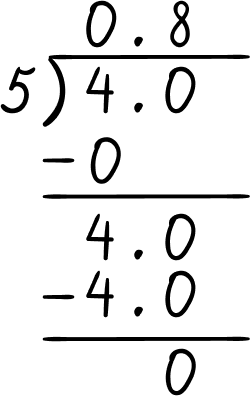0.8 as a percent is 80 \% because you can multiply 0.8 \times 100 = 80

0.5 is not correct because 0.5 < 0.8 \; \bigl( \cfrac{4}{5} \, \bigr)

80 \% is not correct, because 80 \%= 80 \% \; \bigl( \cfrac{4}{5} \, \bigr)

\cfrac{40}{50} \, is not correct, because \cfrac{40}{50}=\cfrac{4}{5}

84\% > 80\% \; \bigl( \cfrac{4}{5} \, \bigr)

5. Put the numbers below in ascending order (least to greatest).

0.3, \; 32 \%, \; \cfrac{31}{100}

0.3, \; \cfrac{31}{100} \, , \; 32 \%32\%, \; \cfrac{31}{100} \, , \; 0.30.3, \; 31\%, \; 0.3232 \%, \; 0.3, \; \cfrac{31}{100}Write the numbers in the same form (make them percents).

Multiply 0.3 by 100 to make it a percent number.

0.3 \times 100 = 30 = 30\%

\cfrac{31}{100} \, is 31 \% because any fraction over 100 can be written as a percent where the numerator represents the percent number.

In order from least to greatest:

30\%, \; 31\%, \; 32\% \; → \; 0.3, \; \cfrac{31}{100} \, , \; 32\%

6. Put the numbers below in descending order (greatest to least).

\cfrac{7}{9} \, , \; 70 \%, \; 0.79

\cfrac{7}{9} \, , \; 0.79 , \; 70 \%70 \%, \; \cfrac{7}{9} \, , \; 0.7970 \% , \; 0.79, \; \cfrac{7}{9}0.79, \; \cfrac{7}{9} \, , \; 70 \%Change the numbers to the same form (make them decimals).

\cfrac{7}{9}= 7\div 9 = 0.\overline{7}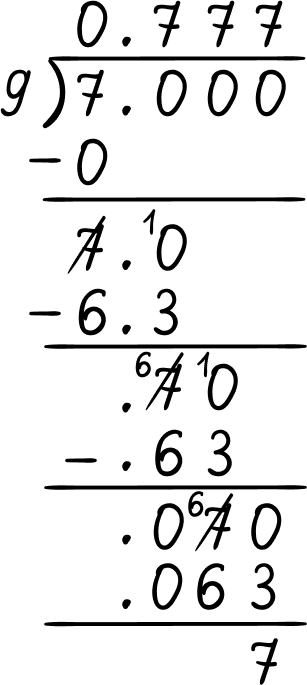0.\overline{7} = 0.77777… rounded to the nearest hundredth is 0.78 .

70 \% is \, \cfrac{70}{100}= 70\div 100 = 0.70

The numbers in descending order are:

0.79, \; 0.78, \; 0.70 \; → \; 0.79, \; \cfrac{7}{9} \, , 0.70

## Comparing fractions, decimals, and percentages FAQs

Is there a way to change a repeating decimal directly into a fraction?

Yes, when you get into 8th grade math and high school math, there is an algebraic process you can use to directly convert a repeating decimal into a fraction.

Can you always move the decimal point two decimal places right when converting a decimal number to a percent?

Yes, when changing a decimal number to a percent you can move the decimal point two places to the right. This is because from the patterns you learned from multiplying by powers of 10, multiplying by 100 is the same as moving the decimal point two places to the right.

0.27 \times 100=27

You can move the decimal point two places to the right.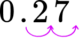Can you always move the decimal point two decimal places left when converting a percent to a decimal?

Yes, using the rules for dividing by powers of ten you can always move the decimal point two decimal places to the left when dividing by 100.

65 \%=\cfrac{65}{100}=65\div 100 = 0.65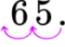Can you convert improper fractions to percents?

Yes, you can convert improper fractions to percents the same way you convert proper fractions to percents.

For example, \cfrac{5}{4} can be converted to a percent by making the denominator 100.

\cfrac{5 \, \times \, 25}{4 \, \times \, 25}=\cfrac{125}{100}= 125\%. The percent will be greater than 100.

## Still stuck?

At Third Space Learning, we specialize in helping teachers and school leaders to provide personalized math support for more of their students through high-quality, online one-on-one math tutoring delivered by subject experts.

Each week, our tutors support thousands of students who are at risk of not meeting their grade-level expectations, and help accelerate their progress and boost their confidence.